# Getting started with ‘ggautomap’

``````library(nswgeo)
library(ggautomap)
library(ggplot2)
library(dplyr, warn.conflicts = FALSE)``````

This article provides some recipes for plots that might be of interest. These examples use map data from the `{nswgeo}` package.

Many of the examples use the same example dataset modelled after the structure of a linelist: rows are distinct events, and they can have a `type` of `A` or `B`. Each event is associated with a location described at different granularities by the `postcode`, `lga`, and `lhd` columns.

``````head(covid_cases_nsw)
#> # A tibble: 6 × 5
#>   postcode lga       lhd                 year type
#>   <chr>    <chr>     <chr>              <int> <chr>
#> 1 2427     Mid-Coast Hunter New England  2022 B
#> 2 2761     Blacktown Western Sydney      2021 A
#> 3 2426     Mid-Coast Hunter New England  2022 B
#> 4 2148     Blacktown Western Sydney      2022 B
#> 5 2768     Blacktown Western Sydney      2021 A
#> 6 2766     Blacktown Western Sydney      2021 B``````

You need to specify which column has the feature by setting the `location` aesthetic. This example has three different columns of locations for different feature types; your dataset only needs to have one of these.

In general you’ll start with `geom_boundaries()` to draw the base map. This geom needs to be told which `feature_type` you’re after (e.g. `"nswgeo.lga"` for LGAs). All of the summary geoms of `ggautomap` can then be used to draw your data.

## Scatter

``````covid_cases_nsw %>%
ggplot(aes(location = lga)) +
geom_boundaries(feature_type = "nswgeo.lga") +
geom_geoscatter(aes(colour = type), sample_type = "random", size = 0.5) +
coord_automap(feature_type = "nswgeo.lga", xlim = c(147, 153), ylim = c(-33.7, -29)) +
guides(colour = guide_legend(override.aes = list(size = 1))) +
theme_void()``````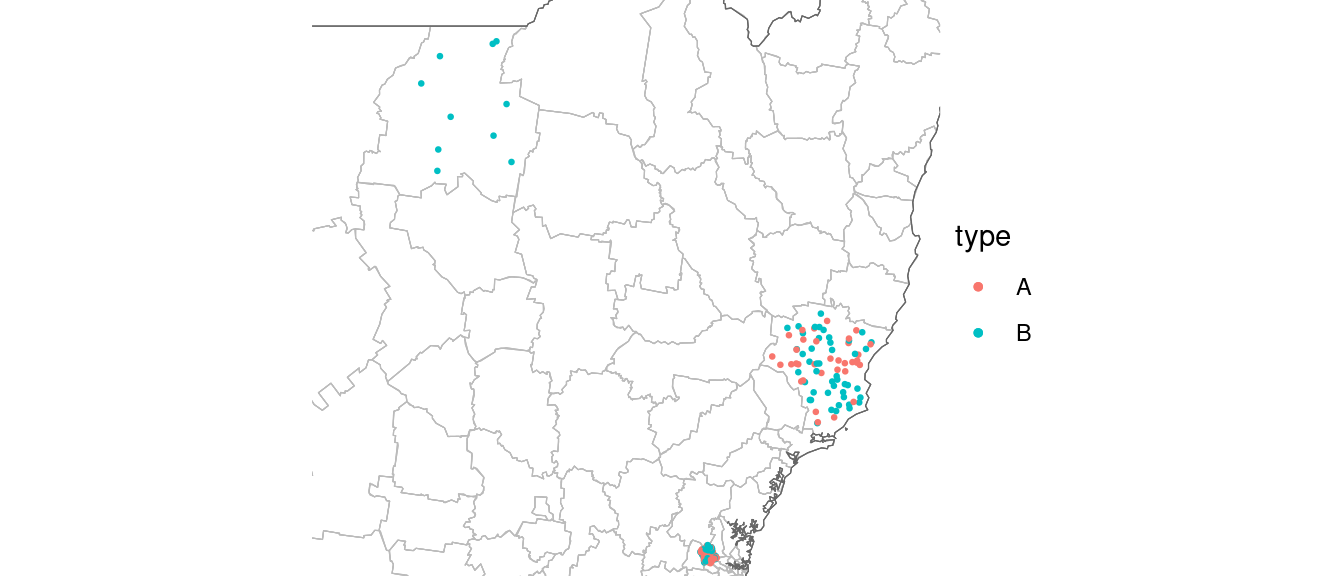Points are drawn at random within the boundaries of their location.

## Insets

To show a zoomed in part of the map as an inset, you can configure an inset and provide it to each relevant geom. The geoms in this package are all inset-aware. See `{ggmapinset}` for details.

``````covid_cases_nsw %>%
ggplot(aes(location = lga)) +
geom_boundaries(feature_type = "nswgeo.lga") +
geom_geoscatter(aes(colour = type), size = 0.5) +
geom_inset_frame() +
coord_automap(feature_type = "nswgeo.lga", inset = configure_inset(
centre = "Blacktown", radius = 40, units = "km",
scale = 7, translation = c(400, -100)
)) +
theme_void()``````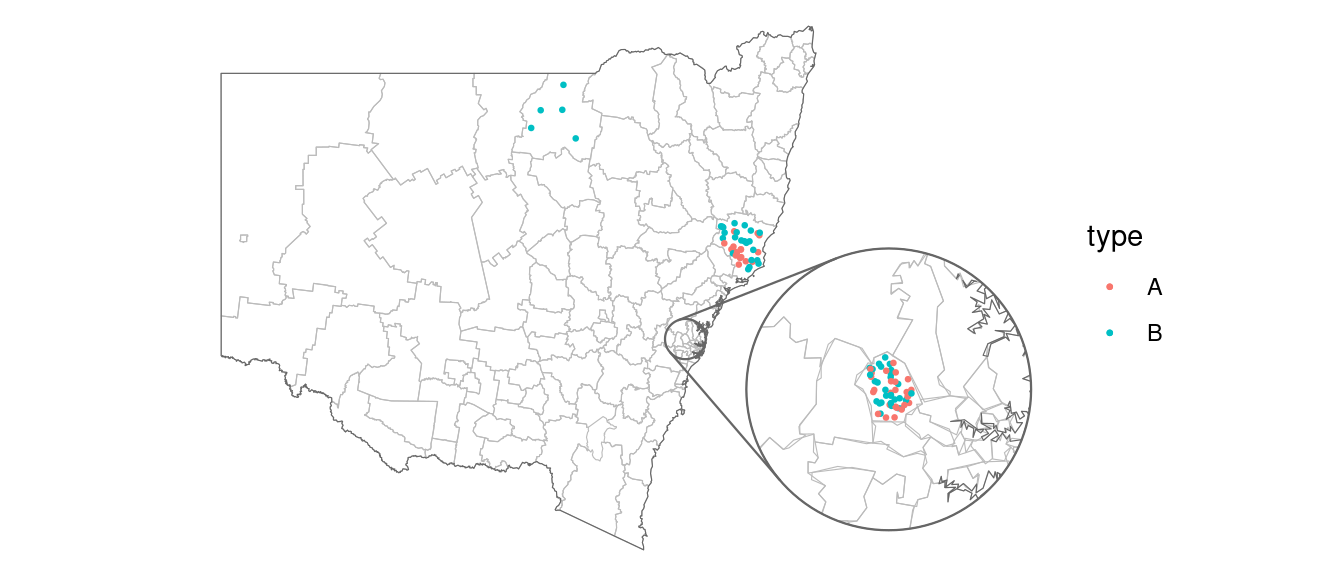## Packed points

This next example uses `geom_centroids()` to place the points in a packed circle in the centre of each feature. It also shows how you can fine-tune the plot with the usual `{ggplot2}` functions.

``````covid_cases_nsw %>%
dplyr::filter(year >= 2021) %>%
ggplot(aes(location = lhd)) +
geom_boundaries(feature_type = "nswgeo.lhd") +
geom_centroids(aes(colour = type), position = position_circle_repel_sf(scale = 35), size = 1) +
geom_inset_frame() +
coord_automap(feature_type = "nswgeo.lhd", inset = configure_inset(
centre = "Sydney", radius = 80, units = "km", feature_type = "nswgeo.lhd",
scale = 6, translation = c(650, -100)
)) +
facet_wrap(vars(year)) +
labs(x = NULL, y = NULL) +
theme_void() +
theme(strip.text = element_text(size = 12))``````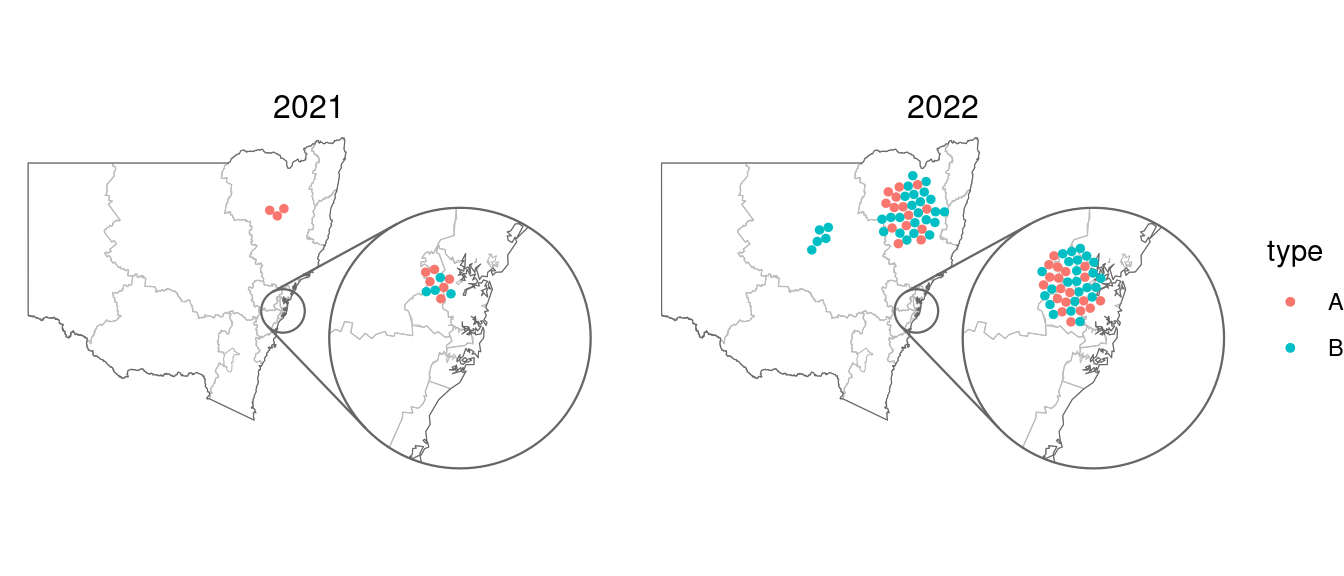## Choropleths

If your data has multiple rows for each location (such as our example dataset where the rows are disease cases) then you can use `geom_choropleth()` to aggregate these into counts.

``````covid_cases_nsw %>%
ggplot(aes(location = lhd)) +
geom_choropleth() +
geom_boundaries(
feature_type = "nswgeo.lhd", colour = "black", linewidth = 0.1,
outline.aes = list(colour = NA)
) +
geom_inset_frame() +
coord_automap(feature_type = "nswgeo.lhd", inset = configure_inset(
centre = "Western Sydney", radius = 60, units = "km",
scale = 5, translation = c(400, -100)
)) +
scale_fill_steps(low = "#e6f9ff", high = "#00394d", n.breaks = 5, na.value = "white") +
theme_void()``````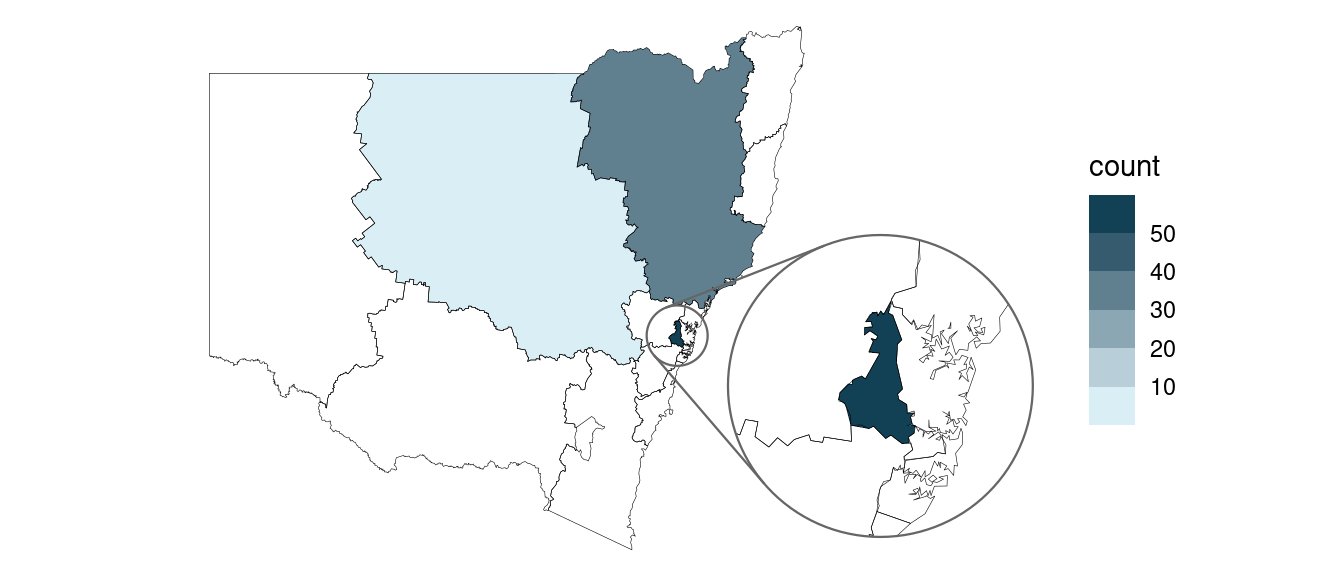On the other hand, if your dataset has only one row per location and there is an existing column that you’d like to map to the `fill` aesthetic, then instead use `geom_sf_inset(..., stat = "automap")`:

``````summarised_data <- data.frame(
lhd = c("Western Sydney", "Sydney", "Far West", "Mid North Coast", "South Western Sydney"),
cases = c(250, 80, 20, NA, 100)
)

summarised_data %>%
ggplot(aes(location = lhd)) +
geom_sf_inset(aes(fill = cases), stat = "automap", colour = NA) +
geom_boundaries(
feature_type = "nswgeo.lhd", colour = "black", linewidth = 0.1,
outline.aes = list(colour = NA)
) +
geom_inset_frame() +
coord_automap(feature_type = "nswgeo.lhd", inset = configure_inset(
centre = "Western Sydney", radius = 60, units = "km",
scale = 3.5, translation = c(350, 0)
)) +
scale_fill_gradient(low = "#e6f9ff", high = "#00394d", na.value = "grey90") +
theme_void()``````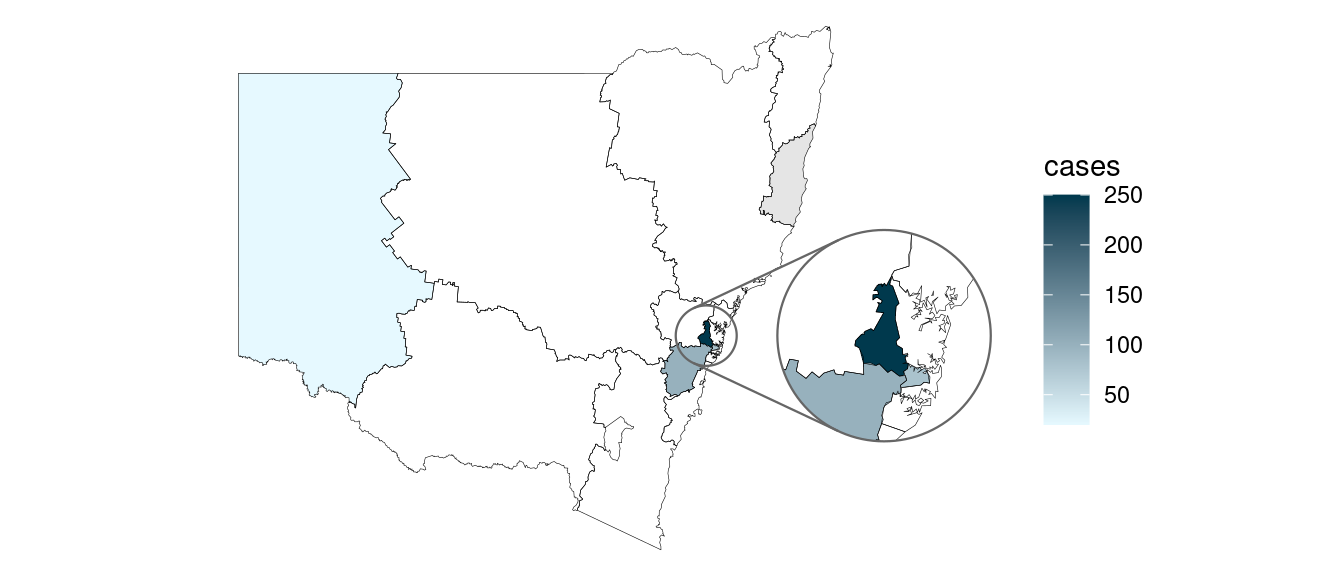## Positioning text

These examples give some different ways of placing text, accounting for possible insets.

``````covid_cases_nsw %>%
ggplot(aes(location = lhd)) +
geom_choropleth() +
geom_boundaries(feature_type = "nswgeo.lhd") +
geom_inset_frame() +
geom_sf_label_inset(aes(label = lhd),
stat = "automap_coords",
data = ~ dplyr::slice_head(.x, by = lhd)
) +
coord_automap(feature_type = "nswgeo.lhd", inset = configure_inset(
centre = "Western Sydney", radius = 60, units = "km",
scale = 3.5, translation = c(350, 0)
)) +
labs(x = NULL, y = NULL) +
theme_void()``````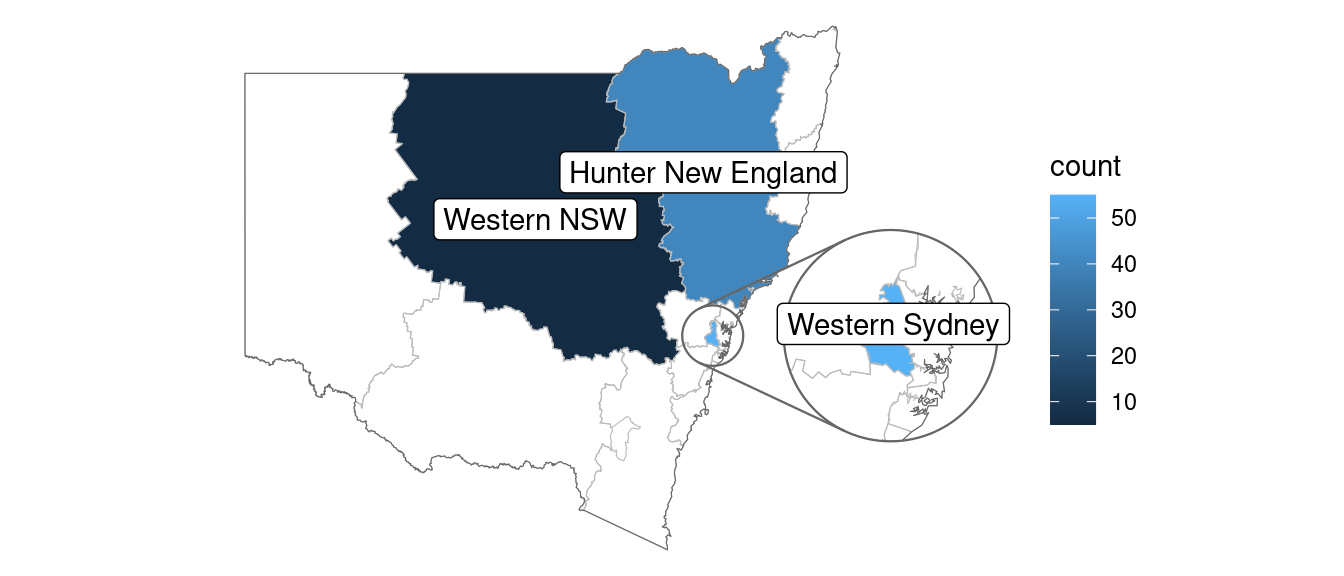The repulsive labels from `{ggrepel}` can be used; they just require a bit of massaging since they don’t natively understand the spatial data. Note that you may also wish to use `point.padding = NA` to disable the default repulsion caused by the labelled points, which is good for labelling scatter plots but often doesn’t make sense in mapping contexts.

``````library(ggrepel)

# label all features that have data
covid_cases_nsw %>%
ggplot(aes(location = lhd)) +
geom_choropleth() +
geom_boundaries(feature_type = "nswgeo.lhd") +
geom_inset_frame() +
geom_label_repel(
aes(
x = after_stat(x_inset),
y = after_stat(y_inset),
label = lhd
),
stat = "automap_coords",
nudge_x = 3,
nudge_y = 1,
data = ~ dplyr::slice_head(.x, by = lhd)
) +
scale_fill_distiller(direction = 1) +
coord_automap(feature_type = "nswgeo.lhd", inset = configure_inset(
centre = "Western Sydney", radius = 60, units = "km",
scale = 3.5, translation = c(350, 0)
)) +
labs(x = NULL, y = NULL) +
theme_void()``````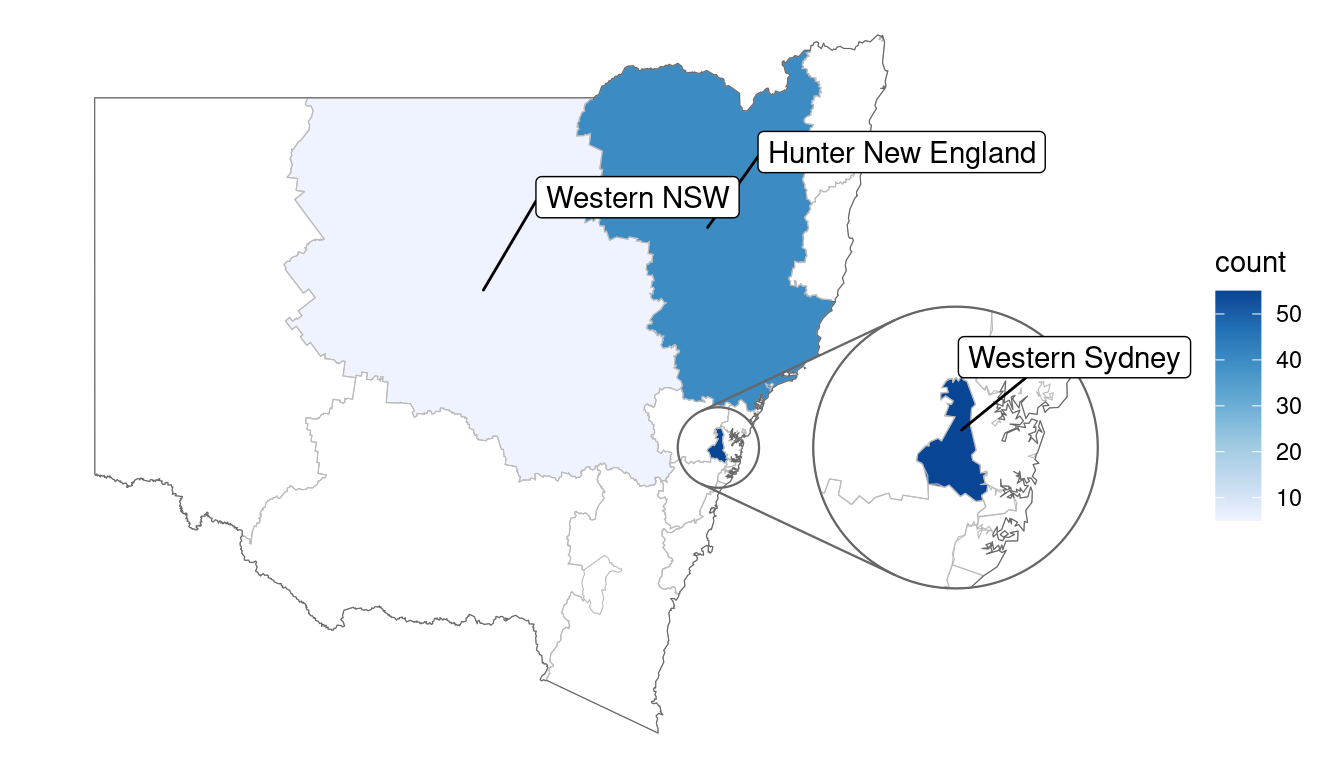``````
# label all features in the map regardless of data, hiding visually overlapping labels
covid_cases_nsw %>%
ggplot(aes(location = lhd)) +
geom_choropleth() +
geom_boundaries(feature_type = "nswgeo.lhd") +
geom_inset_frame() +
geom_label_repel(
aes(
x = after_stat(x_inset),
y = after_stat(y_inset),
geometry = geometry,
label = lhd_name
),
stat = "sf_coordinates_inset",
data = cartographer::map_sf("nswgeo.lhd"),